# Trigonometric chart

Trigonometric chart

Emma is standing in the balcony of her house.

She is looking down at the flower pot placed in the balcony of another house.You can observe a right-angled triangle in this situation.

Emma says that if she knows the height at which she is standing, she can find the distance between both the buildings.

Do you agree with her?

We can find the heights or distances using some mathematical techniques which fall under a branch of mathematics called "Trigonometry."

In this short lesson, we will learn about trigonometry, values in the trigonometric chart, and trigonometric tracer on unit circle.

## Lesson Plan

 1 What Does Trigonometry Formula Refer To? 2 Important Notes on Trigonometry 3 Solved Examples on Trigonometric Chart 4 Tips and Tricks on Trigonometric Chart 5 Interactive Questions on Trigonometric Chart

## What Does Trigonometry Formula Refer To?

Look at the right-angled triangle $$ABC$$ shown below.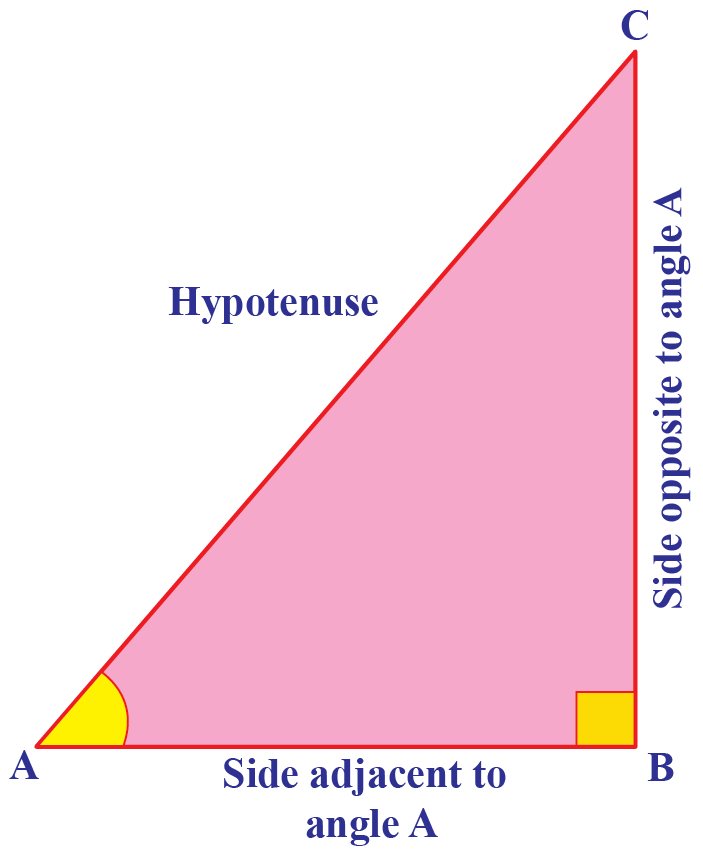$$AC$$ is the hypotenuse.

The side $$AB$$ is the extended portion of $$\angle A$$.

Hence, we call it as the "side adjacent to $$\angle A$$".

The side $$BC$$ faces $$\angle A$$.

Hence, we call it the "side opposite to $$\angle A$$".

### Trigonometric Ratios

We will now define some ratios involving the sides of the triangle $$ABC$$.

 $$\sin{A}=\dfrac{\text{Side Opposite to } \angle A}{\text{Hypotenuse}}=\dfrac{BC}{AC}$$
 $$\cos{A}=\dfrac{\text{Side Adjacent to } \angle A}{\text{Hypotenuse}}=\dfrac{AB}{AC}$$
 $$\tan{A}=\dfrac{\text{Side Opposite to } \angle A}{\text{Side Adjacent to }\angle A}=\dfrac{BC}{AB}$$
 $$\csc{A}=\dfrac{1}{\sin A}=\dfrac{AC}{BC}$$
 $$\sec{A}=\dfrac{1}{\cos A}=\dfrac{AC}{AB}$$
 $$\cot{A}=\dfrac{1}{\tan A}=\dfrac{AB}{BC}$$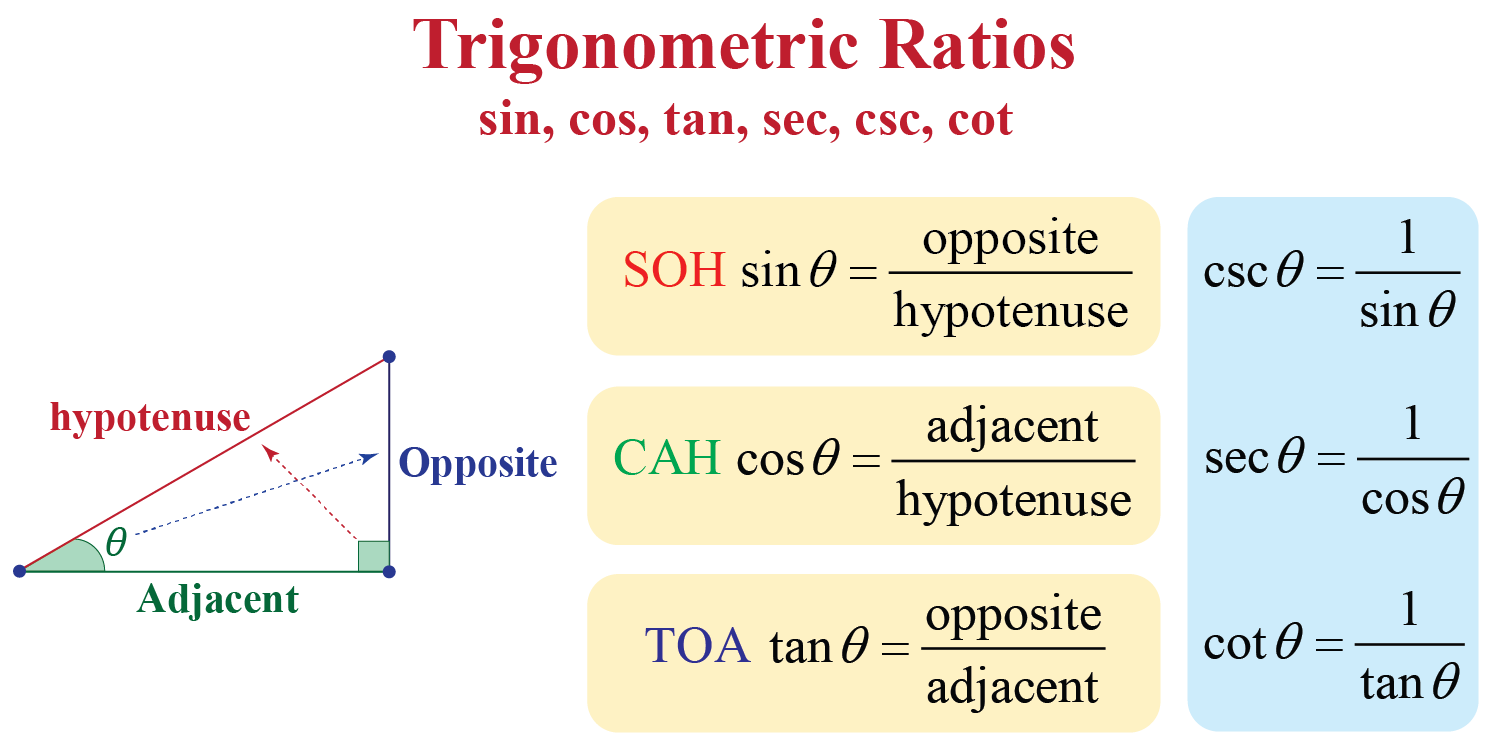## Trigonometric Chart

You are already familiar with construction of angles like $$30^{\circ}$$, $$45^{\circ}$$, $$60^{\circ}$$, and $$90^{\circ}$$.

We will now see the values in the trigonometric chart for these angles.

$$\angle A$$ $$0^{\circ}$$ $$30^{\circ}$$ $$45^{\circ}$$ $$60^{\circ}$$ $$90^{\circ}$$
$$\sin {A}$$ 0 $$\dfrac{1}{2}$$ $$\dfrac{1}{\sqrt{2}}$$ $$\dfrac{\sqrt{3}}{2}$$ 1
$$\cos {A}$$ 1 $$\dfrac{\sqrt{3}}{2}$$ $$\dfrac{1}{\sqrt{2}}$$ $$\dfrac{1}{2}$$ 0
$$\tan {A}$$ 0 $$\dfrac{1}{\sqrt{3}}$$ 1 $$\sqrt{3}$$ Not defined
$$\csc {A}$$ Not defined 2 $$\sqrt{2}$$ $$\dfrac{2}{\sqrt{3}}$$ 1
$$\sec {A}$$ 1 $$\dfrac{2}{\sqrt{3}}$$ $$\sqrt{2}$$ 2 Not defined
$$\cot {A}$$ Not defined $$\sqrt{3}$$ 1 $$\dfrac{1}{\sqrt{3}}$$ 0

Experiment with the simulation below to determine the values of all trigonometric functions for distinct angles and observe the values being plotted on the graphs.

## What Are the 8 Trigonometric Identities?

An equation becomes an identity when it is true for all the variables involved in it.

Now we will discuss trigonometric identities which are true for all angles involved in it.

 $$\cos^{2}{A}+\sin^{2}{A}=1$$
 $$1+\tan^{2}{A}=\sec^{2}{A}$$
 $$1+\cot^{2}{A}=\csc^{2}{A}$$
 $$\cos{(2A)}=2\cos^{2}{A}-1=1-2\sin^{2}{A}$$
 $$\sin{(2A)}=2\cos{A}\sin{A}$$
 $$\tan{(2A)}=\dfrac{2\tan{A}}{1-\tan^{2}{A}}$$
 $$\cos{(2A)}=\cos^{2}{A}-\sin^{2}{A}$$
 $$\sin{(2A)}=\dfrac{2\tan{A}}{1+\tan^{2}{A}}$$

Apart from these identities, we have a few more formulas which are helpful for you in solving trigonometric problems.

##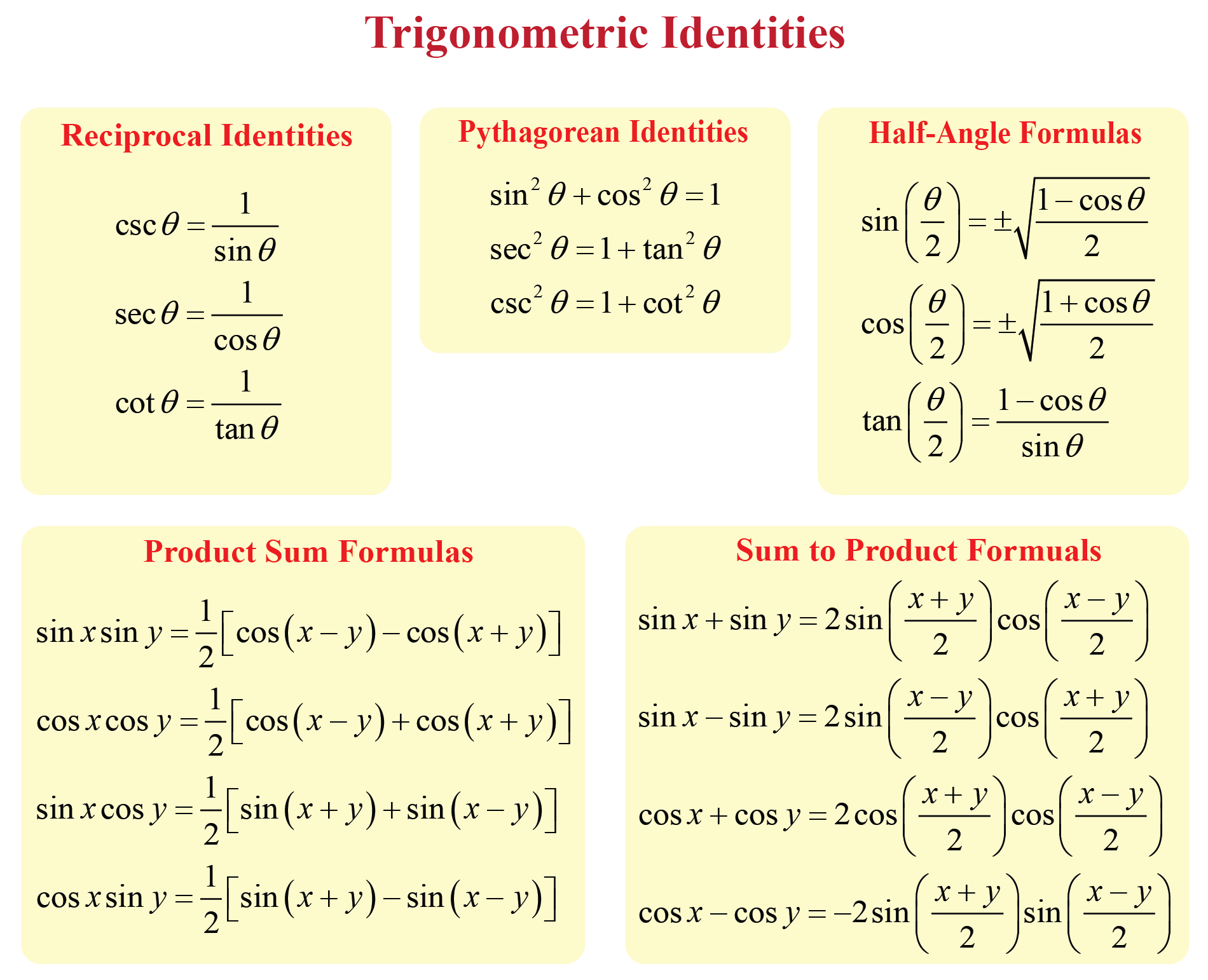Example on Trigonometry

Trigonometry is a branch of mathematics that is used to solve geometric problems involving triangles.

### Example

Let's assume that $$ABC$$ is a right-angled triangle with $$\tan{A}=\dfrac{4}{3}$$.

Let's find all other trigonometric ratios of $$\angle A$$.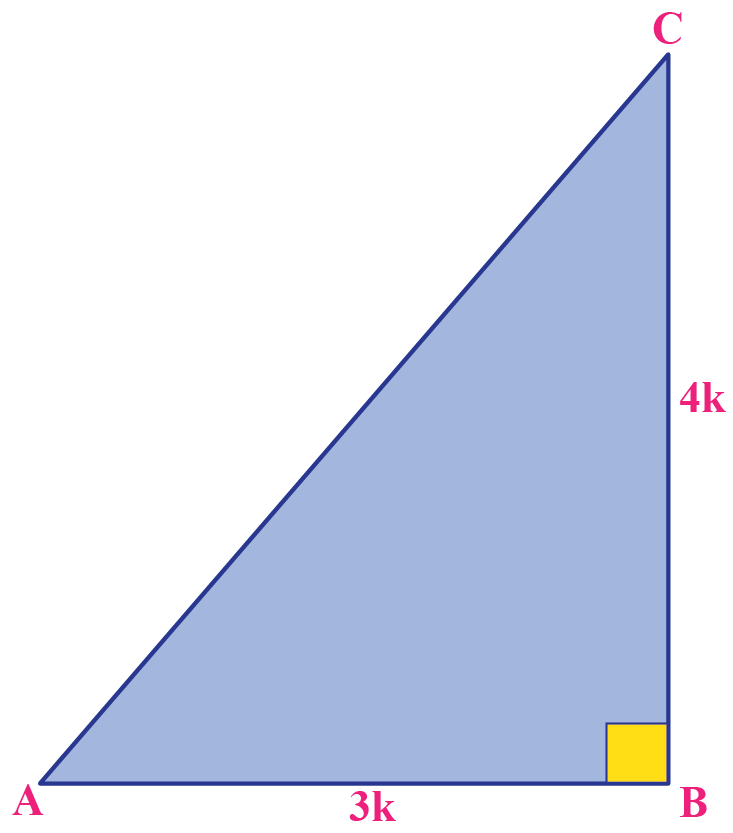We know that $$\tan{A}=\dfrac{BC}{AB}=\dfrac{4}{3}$$.

Hence, we can write $$BC=4k$$ and $$AB=3k$$, where $$k$$ is a positive integer.

Using Pythagoras theorem,

\begin{align}(AC)^{2}&=(AB)^{2}+(BC)^{2}\\&=9k^{2}+16k^{2}\\&=25k^{2}\end{align}

Thus, $$AC=5k$$.

Other trigonometric ratio are:

$\sin{A}=\dfrac{BC}{AC}=\dfrac{4k}{5k}=\dfrac{4}{5}$

$\cos{A}=\dfrac{AB}{AC}=\dfrac{3k}{5k}=\dfrac{3}{5}$

$\cot{A}=\dfrac{1}{\tan{A}}=\dfrac{3}{4}$

$\csc{A}=\dfrac{1}{\sin{A}}=\dfrac{5}{4}$

$\sec{A}=\dfrac{1}{\cos{A}}=\dfrac{5}{3}$

### Trigonometric Tracer on Unit Circle

Here is a trigonometric tracer that shows all the trigonometric functions on a unit circle.

Choose a trigonometric function and observe its relation with the unit circle and see how its graph is traced out over a complete angle.

## What Are the Rules of Trigonometry?

### Signs of Trigonometric Functions

The following table shows the signs of different trigonometric functions in different quadrants.

$$\sin {A}$$ + + - -
$$\cos {A}$$ + - - +
$$\tan {A}$$ + - + -
$$\csc {A}$$ + + - -
$$\sec {A}$$ + - - +
$$\cot {A}$$ + - + -

## Where is Trigonometry Used?

Do you know that trigonometry is one of the most ancient subjects which is studied by scholars all over the world?

The need for trigonometry first arose in astronomy.

In astronomy, it is used to determine the distances from the Earth to the planets and stars.

In geography and navigation, it is used to construct maps. It is also used to find the position of an island in relation to the longitudes and latitudes.

Even today, some of the technologically advanced methods which are used in engineering and physical sciences are based on the concepts of trigonometry.

Trigonometry is also used in the science of seismology to design electric circuits, describe the state of an atom, and predict the heights of tides in the ocean.

## Solved Examples

 Example 1

A contractor wants to install a slide for children in a park.

He designs the slide such that its top is at a height of 5 feet and the angle is $$30^{\circ}$$ with the ground.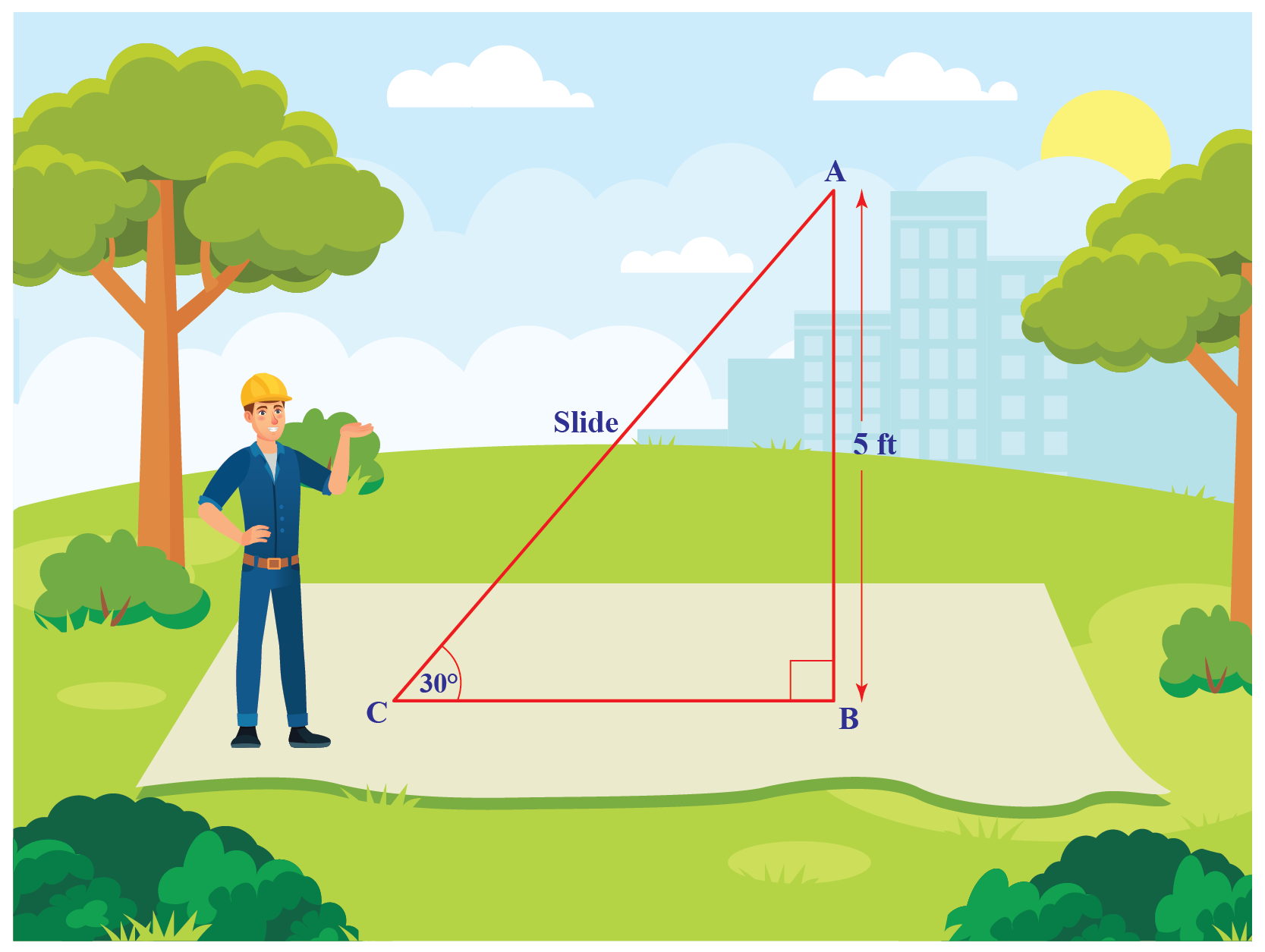Can you determine the length of the slide?

Solution

Let's represent the length of the slide by $$AC$$.

Since the ratio involves the sides $$AB$$ and $$AC$$, we will use the trigonometric ratio $$\sin{30^{\circ}}$$.

\begin{align}\sin{30^{\circ}}&=\dfrac{AB}{AC}\\\dfrac{1}{2}&=\dfrac{5}{AC}\\AC&=10\end{align}

 $$\therefore$$ The length of the slide is 10 feet.
 Example 2

Look at the triangle below.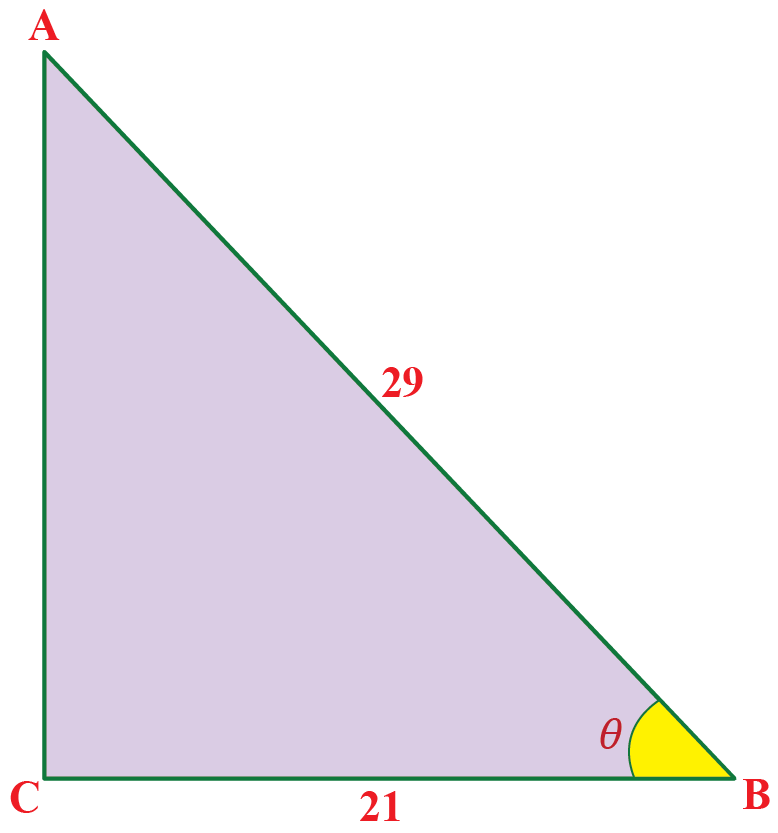The triangle is right-angled at $$C$$ with $$AB=29\;\text{units}$$ and $$BC=21\;\text{units}$$.

Can you verify the trigonometric identity $$\cos^{2}{A}+\sin^{2}{A}=1$$ using these values?

Solution

We will find $$AC$$ using Pythagoras theorem.

\begin{align}AC&=\sqrt{(AB)^{2}-(BC)^{2}}\\&=\sqrt{29^{2}-21^{2}}\\&=\sqrt{400}\\&=20\end{align}

Let's determine the values of $$\sin{\theta}$$ and $$\cos{\theta}$$.

$\sin{\theta}=\dfrac{AC}{AB}=\dfrac{20}{29}$

$\cos{\theta}=\dfrac{BC}{AB}=\dfrac{21}{29}$

Now let's verify the identity.

\begin{align}\cos^{2}{A}+\sin^{2}{A}&=\left(\dfrac{21}{29}\right)^{2}+\left(\dfrac{20}{29}\right)^{2}\\&=\dfrac{400+441}{841}\\&=1\end{align}

 $$\therefore$$ The identity is verified.
 Example 3

Jack is standing on the ground and looking at the top of the tower with the angle of elevation as $$60^{\circ}$$.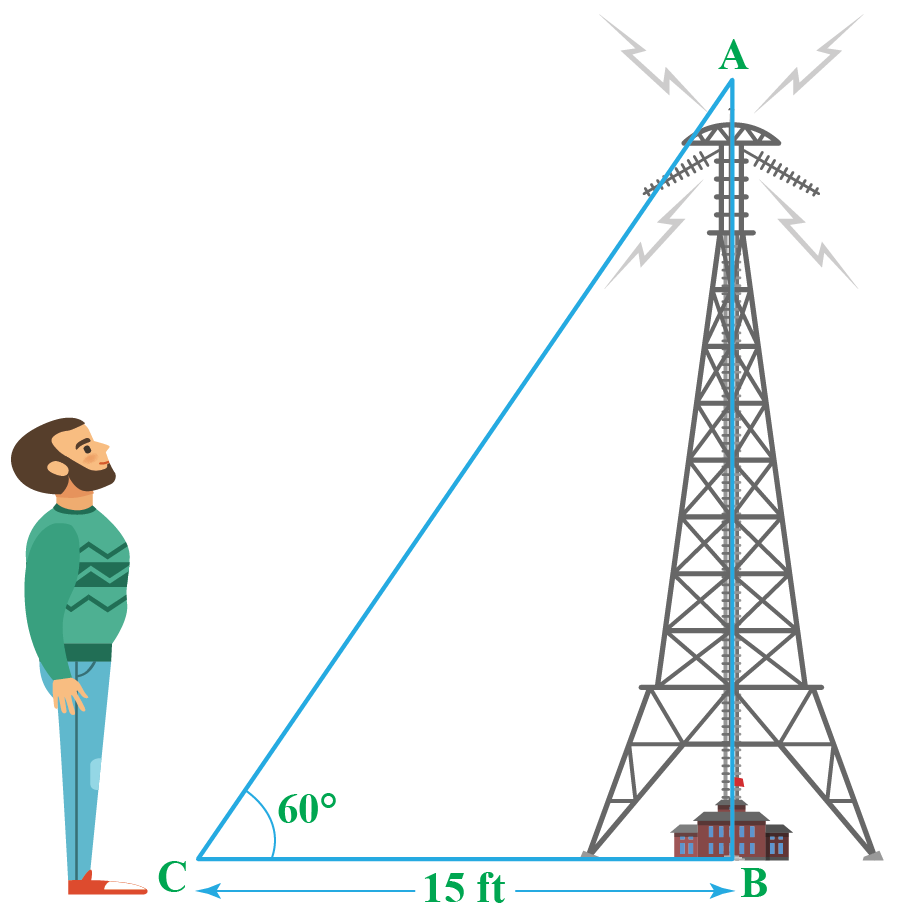If he is standing 15 feet away from the foot of the tower, can you determine the height of the tower?

### Solution

Since the ratio involves the sides $$AB$$ and $$BC$$, we will use the trigonometric ratio $$\tan{60^{\circ}}$$.

\begin{align}\tan{60^{\circ}}&=\dfrac{AB}{BC}\\\sqrt{3}&=\dfrac{AB}{15}\\AB&=15\sqrt{3}\end{align}

 $$\therefore$$ The height of the tower is $$15\sqrt{3}$$ feet.
 Example 4

Rachel drew a triangle right-angled at Q with PQ as 3 units and PR as 6 units.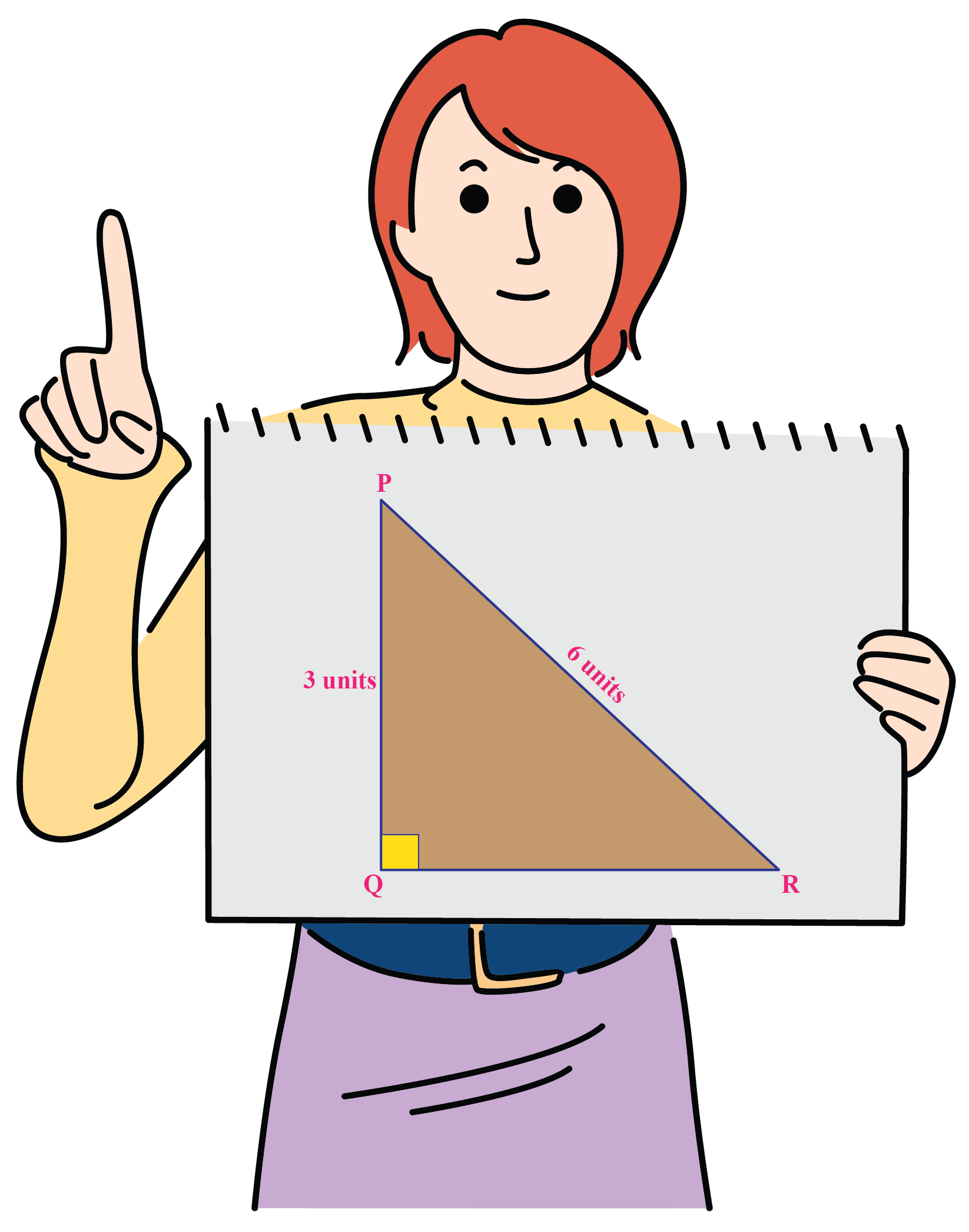Can you determine the angles $$\angle QPR$$ and $$\angle PRQ$$?

Solution

Using sine trigonometric ratio we have,

$\sin{R}=\dfrac{PQ}{PR}=\dfrac{3}{6}=\dfrac{1}{2}$

Sine function takes the value $$\dfrac{1}{2}$$ at $$30^{\circ}$$.

So, $$\angle PRQ=30^{\circ}$$.

Using the angle sum property of triangles,

\begin{align}\angle PRQ+\angle QPR+\angle PQR&=180^{\circ}\\30^{\circ}+90^{\circ}+\angle QPR&=180^{\circ}\\\angle QPR&=180^{\circ}-120^{\circ}\\\angle QPR&=60^{\circ}\end{align}

 $$\therefore$$ The values of angles are $$\angle QPR=60^{\circ}$$, $$\angle PRQ=30^{\circ}$$.

Here are some tips and tricks that will help you solve trigonometric problems.Tips and Tricks
1. The values of trigonometric ratios do not change with the change in the side lengths of the triangle if the angle remains the same.
2. The values of $$\sin{A}$$ and $$\cos{A}$$ is always less than or equal to 1.
3. From the trigonometric chart, you can observe that as $$\angle A$$ increases from $$0^{\circ}$$ to $$90^{\circ}$$, $$\sin{A}$$ increases from 0 to 1 and $$\cos{A}$$ decreases from 1 to 0.
4. $$\sin{A}$$, $$\tan{A}$$, $$\cot{A}$$ and $$\csc{A}$$ are odd functions.
5. $$\cos{A}$$ and $$\sec{A}$$ are even functions.

## Interactive Questions

Here are a few activities for you to practice. Select/type your answer and click the "Check Answer" button to see the result.

## Let's Summarize

This mini-lesson targeted the fascinating concept of the Trigonometric Chart. The math journey around Trigonometric Chart starts with what a student already knows, and goes on to creatively crafting a fresh concept in the young minds. Done in a way that not only it is relatable and easy to grasp, but also will stay with them forever. Here lies the magic with Cuemath.

We hope you enjoyed trying your hand at solving a few interactive questions on the concept of Trigonometric Chart.

At Cuemath, our team of math experts are dedicated to making learning fun for our favorite readers, the students! Through an interactive and engaging learning-teaching-learning approach, the teachers explore all angles of a topic. Be it problems, online classes, doubt sessions, or any other form of relation, it’s the logical thinking and smart learning approach that we, at Cuemath, believe in.

## 1. What are the basic formulas of trigonometry?

The basic formulas of trigonometry are:

• $$\cos^{2}{A}+\sin^{2}{A}=1$$
• $$1+\tan^{2}{A}=\sec^{2}{A}$$
• $$\cot^{2}{A}+1=\csc^{2}{A}$$

## 2. What is the easiest way to learn trigonometry?

Follow the points mentioned below to learn trigonometry in the easiest way.

• Familiarize yourself with the parts of a triangle like a hypotenuse, obtuse angle, and acute angle.
• Learn to make a unit circle which will help you relate all trigonometric functions.
• Remember the six trigonometric functions.
• Practice problems to memorize all the trigonometric identities.

## 3. Who is the father of Trigonometry?

The great mathematician Hipparchus was the founder of trigonometry. Therefore, he is known as the father of trigonometry.

More Important Topics
Numbers
Algebra
Geometry
Measurement
Money
Data
Trigonometry
Calculus
More Important Topics
Numbers
Algebra
Geometry
Measurement
Money
Data
Trigonometry
Calculus
Learn from the best math teachers and top your exams

• Live one on one classroom and doubt clearing
• Practice worksheets in and after class for conceptual clarity
• Personalized curriculum to keep up with school